Courses

# Match the Following, of Moving Charges and Magnetism , Past year Questions JEE Advance JEE Notes | EduRev

## Class 12 Physics 35 Years JEE Mains &Advance Past year Paper

Created by: Abhishek Kapoor

## JEE : Match the Following, of Moving Charges and Magnetism , Past year Questions JEE Advance JEE Notes | EduRev

The document Match the Following, of Moving Charges and Magnetism , Past year Questions JEE Advance JEE Notes | EduRev is a part of the JEE Course Class 12 Physics 35 Years JEE Mains &Advance Past year Paper.
All you need of JEE at this link: JEE

DIRECTIONS (Qs. 1-3) : Each question contains statements given in two columns, which have to be matched. The statements in Column-I are labelled A, B, C and D, while the statements in Column-II are labelled p, q, r and s. Any given statement in Column-I can have correct matching with ONE OR MORE statement(s) in Column-II. The appropriate bubbles corresponding to the answers to these questions have to be darkened as illustrated in the following example : If the correct matches are A-p, s and t; B-q and r; C-p and q; and D-s then the correct darkening of  bubbles will look like the given.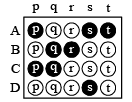Q.1.1. Match the following columns :

 Column I Column II (A) Dielectric ring uniformly charged (p) Constant electrostatic field out of system (B) Dielectric ring uniformly chargedrotating with angular velocity ω (q) Magnetic field strength (C) Constant current in ring i (r) Electric field (induced) (D) i = i0cosωt (s) Magnetic dipole moment

Ans. A-p; B-q, s; C-q, s; D-q, r, s

Solution. (A) Charge on ring will create electric field which is time independent.

(B) The rotating charge is like a current. This will create a magnetic field and a magnetic moment.

(C) Since net charge is zero there will be no time independent electric field. The current produces magnetic field and magnetic moment.

(D) A changing magnetic field will be produced. This will create a induced electric field. Also a changing magnetic moment will be produced.

Q.  Each question contains statements given in two columns, which have to be matched. The statements in Column-I are labelled A, B, C and D, while the statements in Column-II are labelled p, q, r and s. Any given statement in Column-I can have correct matching with ONE OR MORE statement(s) in Column-II. The appropriate bubbles corresponding to the answers to these questions have to be darkened as illustrated in the following example : If the correct matches are A-p, s and t; B-q and r; C-p and q; and D-s then the correct darkening of  bubbles will look like the given.Q.2.Column I gives certain situations in which a straight  metallic wire of resistance R is used and Column II gives some resulting effects. Match the statements in Column I with the statements in Column II and indicate your answer by darkening appropriate bubbles in the 4 × 4 matrix given in the ORS.

 Column I Column II (A) A charged capacitor is connected to the ends of the wire (p) A constant current flows through the wire (B) The wire is moved perpendicular to its length with a constant velocity in a uniform magnetic field perpendicular to the plane of motion (q) Thermal energy is generated  in the wire (C) The wire is placed in a constant electric field that has a direction along the length of the wire (r) A constant potential difference develops between the ends of the wire (D) A battery of constant emf is connected to the ends of the wire. (s) charges of constant magnitude appear at the ends of the wire

Ans. A-q; B-r, s; C-s; D-p, q, r

Solution. A : q

Reason : When a charged capacitor is connected to the ends of the wire, a variable current (decreasing in magnitude with time) passes through the wire (shown as resistor) and thermal energy is generated. The potential difference across the wire also decreases with time. The charge on the capacitor plate also decreases with time.

B : r. s

Reason : e = Blv

When B, l,v are constant, e is constant

⇒ A constant potential difference develops across the ends of the wire and charges of constant magnitude appear at the ends of the wire.

C : s

Reason : The free electrons move under the influence of electric field opposite to the direction of electric field. This movement of e continues till the electric field inside the wire is zero.

⇒ Changes of constant magnitude appear at the ends of the wire.

D : p, q, r

Reason : Since, E, R are constant, a constant current flows in the wire. Due to heating effect of current, thermal energy is generated in the wire. Also a constant potential difference develops between the ends of the wire.

Each question contains statements given in two columns, which have to be matched. The statements in Column-I are labelled A, B, C and D, while the statements in Column-II are labelled p, q, r and s. Any given statement in Column-I can have correct matching with ONE OR MORE statement(s) in Column-II. The appropriate bubbles corresponding to the answers to these questions have to be darkened as illustrated in the following example : If the correct matches are A-p, s and t; B-q and r; C-p and q; and D-s then the correct darkening of  bubbles will look like the given.Q.3. Two wires each carrying a steady current I are shown in four configurations in Column I. Some of the resulting effects are described in Column II. Match the statements in Column I with the statements in column II and indicate your answer by darkening appropriate  bubbles in the 4 × 4 matrix given in the ORS.

 Column I Column II (A) Point P is situated midway between the wires.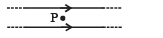(p) The magnetic fields (B) at P due to the currents (B) Point P is situated  at the mid-point of the line joining the centers of the circular wires, which have same radii.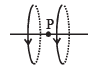(q) The magnetic fields (B) at P due to the currents in the wires are in opposite directions. (C) Point P is situated at the mid-point of the line joining the centers of the circular wires, which have same radii.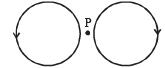(r) There is no magnetic field at P. (D) Point P is situated at the common center of the wires.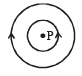(s) The wires repel each other.

Ans. A-q, r; B-p; C-q, r; D-q, s

Solution. A : q, r

Reason : The magnetic field at P due to current flowing in AB is perpendicular to the plane of paper acting vertically downward. And the magnetic field at P due to current flowing in CD is perpendicular to the plane of paper acting vertically upwards.

Therefore, q is correct.

As P is the mid point, the two magnetic fields, cancel out each other. Therefore, r is correct.

B : p

Reason : The magnetic field at P due to current in loop A is along the axial line towards right. Similarly, the magnetic field at P due to current in loop B is also along the axial line towards right.

C : q, r

Reason : The magnetic field due to current in loop A at P is equal and opposite to the magnetic field due to current in loop B at P.

D : q, s

Reason : The direction of magnetic field at P due to current in loop A is perpendicular to the plane of paper directed vertically upwards.

The direction of magnetic field at P due to current in loop B is perpendicular to the plane of paper directed vertically downward.

Since the current are in opposite direction the wires repel each other. But net force on each wire is zero.

Offer running on EduRev: Apply code STAYHOME200 to get INR 200 off on our premium plan EduRev Infinity!

42 docs|19 tests

,

,

,

,

,

,

,

,

,

,

,

,

,

,

,

,

,

,

,

,

,

,

,

,

,

,

,

;